Some days ago Thierry Wasylczenko blogged about 12 of his favorite JS libs. On of the libs is js-sequence-diagrams that I did know. By using the library you can draw sequence diagrams in a browser. I really like the hand drawn theme of the tool that draw all the diagrams like they are sketched with a pen. Here is an example of the theme: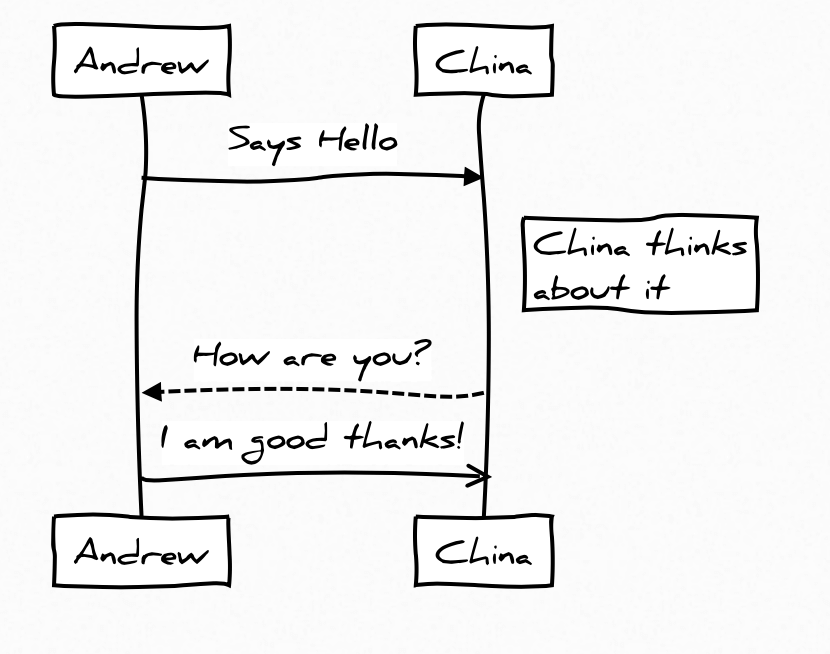After having a look at the source code of js-sequence-diagrams I found the methods that render all the hand drawn lines. Cubic curves are used here and the control points of the curves are calculated by using random values. These two control points define the bend of the curve as you can see in the following picture: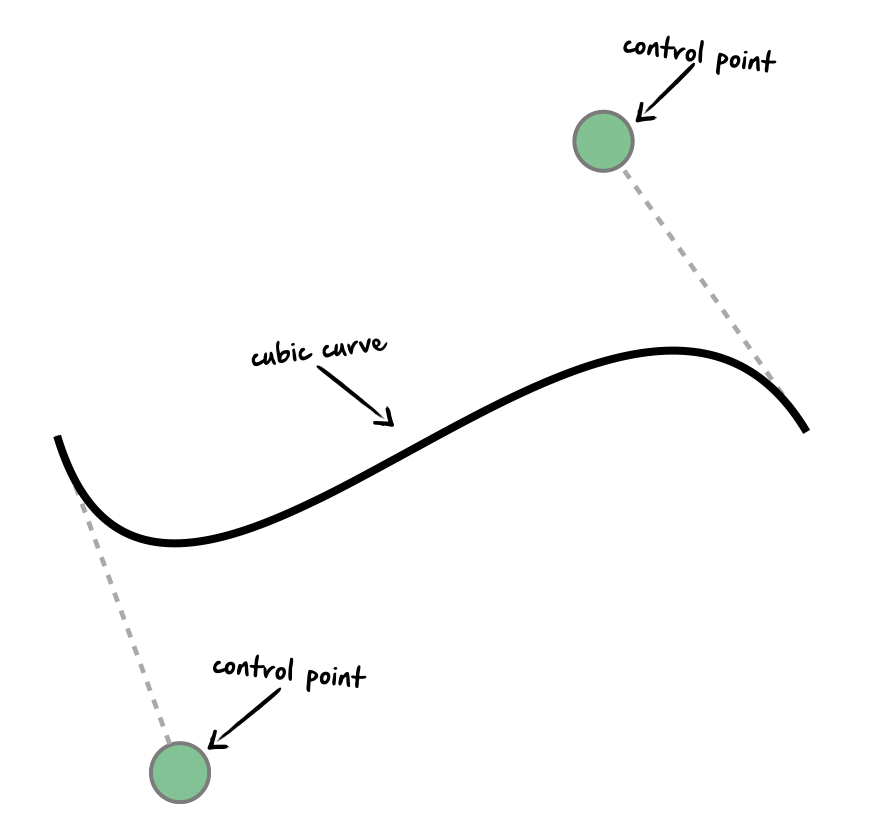Once I’ve seen this I wanted to try if I can do the same with JavaFX :)

Thankfully JavaFX contains support for cubic curves. The Path shape can contain curves that can be defined by the CubicCurveTo class. By using this class you can create lines and shapes that look like hand drawn onces by using the same algorithm as in js-sequence-diagrams. I created a simple test class that draws some arrows, lines and rectangles by using this algorithm. Here are some results: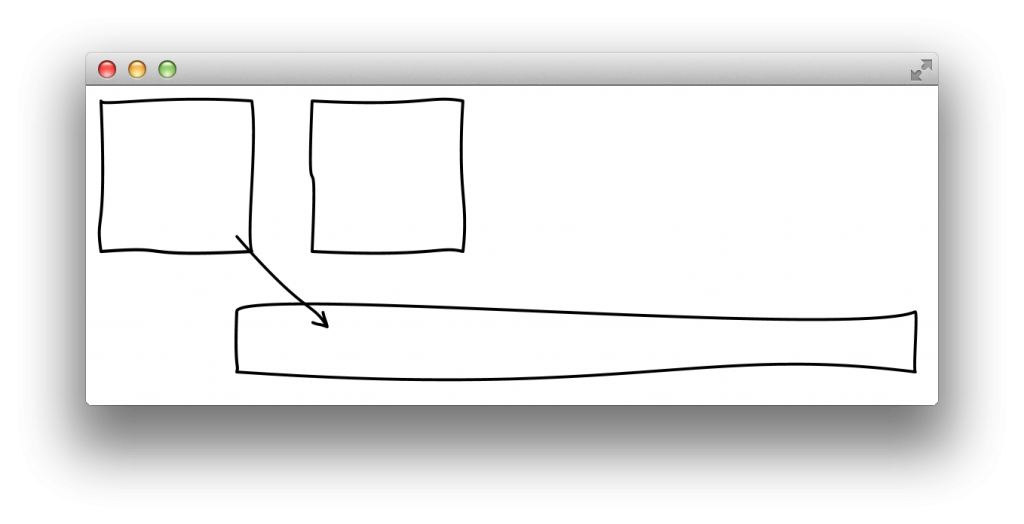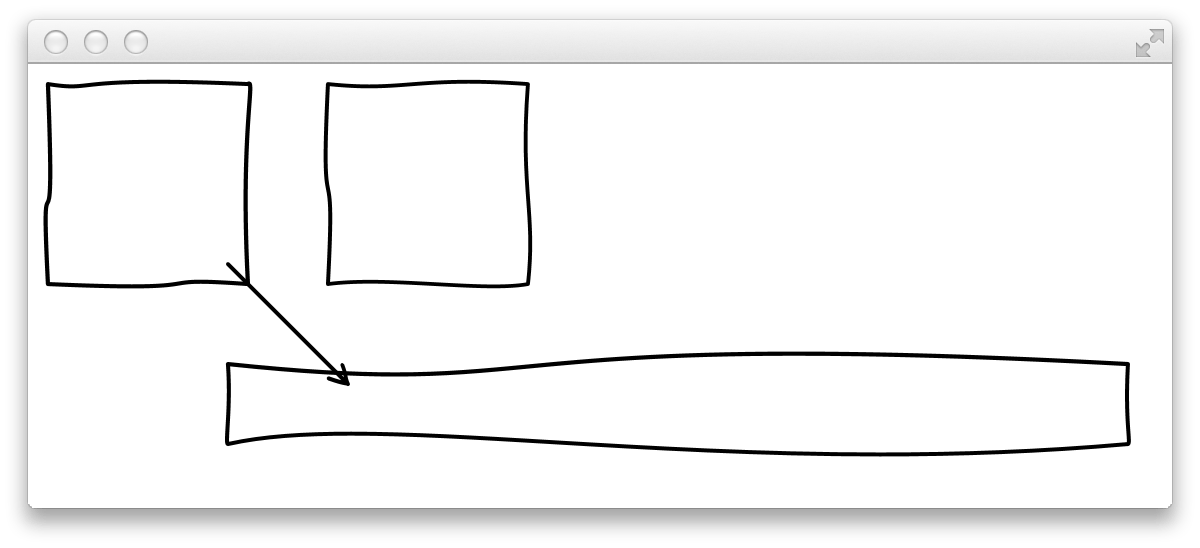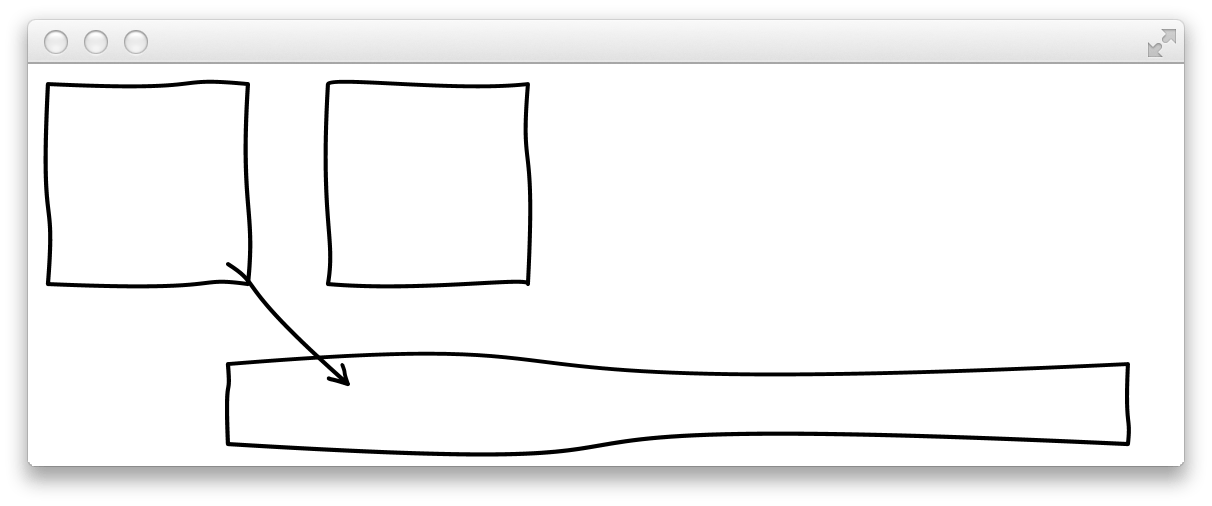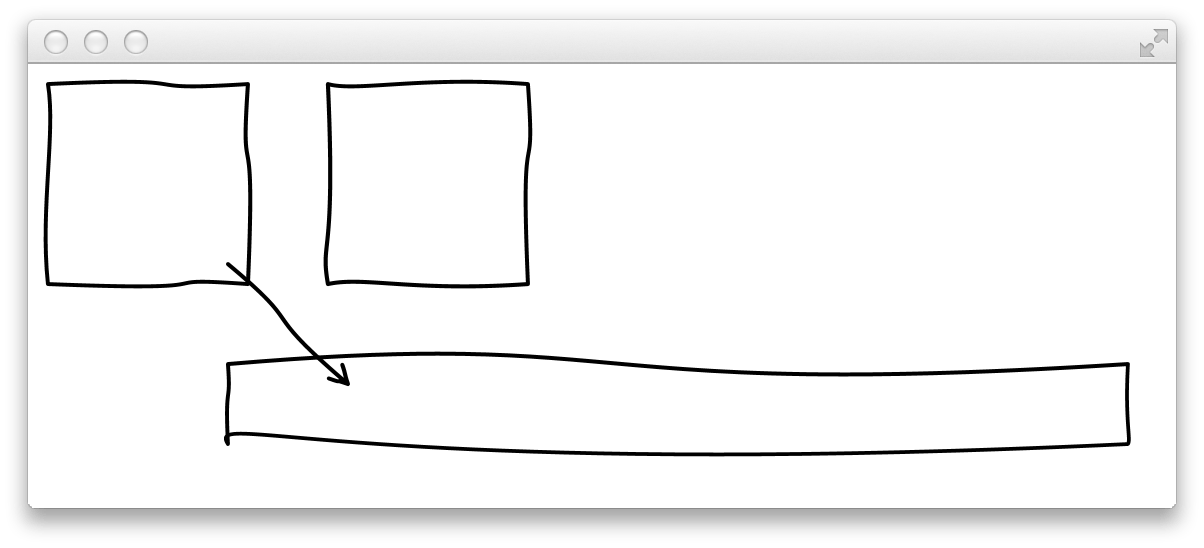As you can see in the picture the shapes look different in each of them. This is caused by the random values that are part of the algorithm. If you want to try this here is my one class gist that contains all the code: